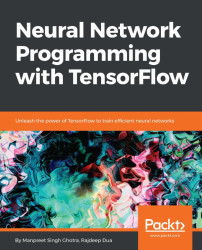•#### Neural Network Programming with Tensorflow#### Overview of this book

If you're aware of the buzz surrounding the terms such as "machine learning," "artificial intelligence," or "deep learning," you might know what neural networks are. Ever wondered how they help in solving complex computational problem efficiently, or how to train efficient neural networks? This book will teach you just that. You will start by getting a quick overview of the popular TensorFlow library and how it is used to train different neural networks. You will get a thorough understanding of the fundamentals and basic math for neural networks and why TensorFlow is a popular choice Then, you will proceed to implement a simple feed forward neural network. Next you will master optimization techniques and algorithms for neural networks using TensorFlow. Further, you will learn to implement some more complex types of neural networks such as convolutional neural networks, recurrent neural networks, and Deep Belief Networks. In the course of the book, you will be working on real-world datasets to get a hands-on understanding of neural network programming. You will also get to train generative models and will learn the applications of autoencoders. By the end of this book, you will have a fair understanding of how you can leverage the power of TensorFlow to train neural networks of varying complexities, without any hassle. While you are learning about various neural network implementations you will learn the underlying mathematics and linear algebra and how they map to the appropriate TensorFlow constructs.
Title PageCreditswww.PacktPub.comCustomer FeedbackPrefaceFree Chapter
Maths for Neural NetworksDeep Feedforward NetworksOptimization for Neural NetworksConvolutional Neural NetworksRecurrent Neural NetworksGenerative ModelsDeep Belief NetworkingAutoencodersResearch in Neural NetworksGetting started with TensorFlow## Types of optimizers

First, we look at the high-level categories of optimization algorithms and then dive deep into the individual optimizers.

First order optimization algorithms minimize or maximize a loss function using its gradient values concerning the parameters. The popularly used First order optimization algorithm is gradient descent. Here, the first order derivative tells us whether the function is decreasing or increasing at a particular point. The first order derivative gives us a line which is tangential to a point on its error surface.

### Note

The derivative for a function depends on single variables, whereas a gradient for a function depends on multiple variables.

Second order optimization algorithms use the second order derivative, which is also known as Hessian, to minimize or maximize the given loss function. Here, the Hessian is a matrix of second order partial derivatives. The second derivative is costly to compute. Hence, it's not used much. The second order derivative indicates...